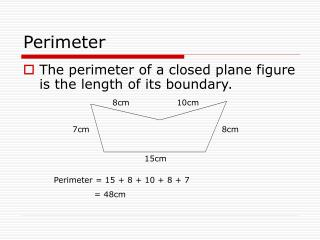DownloadDownload PresentationPerimeter

# Perimeter

Download Presentation## Perimeter

- - - - - - - - - - - - - - - - - - - - - - - - - - - E N D - - - - - - - - - - - - - - - - - - - - - - - - - - -
##### Presentation Transcript

1. Perimeter • The perimeter of a closed plane figure is the length of its boundary. 8cm 10cm 7cm 8cm 15cm Perimeter = 15 + 8 + 10 + 8 + 7 = 48cm

2. Perimeter • Square • Triangles • Rectangle b=4cm b c L= 8cm a L=6cm P = 8 + 5 + 8 + 5 P = 6 + 6 + 6 + 6 P = a + b + c P = l + b + l + b = 2(l + b) P = l + l + l + l = 4l or 4s

3. Area • Area is the space within the boundary of a figure.

4. Area of parallelogram • Area of parallelogram = base x height height base

5. Area of a triangle • Area = ½ x base x height h h h b b b

6. Area of trapezium • Area of trapezium ABCD = area of ΔABC + area of ΔACD = (½ x BC x AH) + (½ x AD x CK) = ½ x AH x (BC + AD) = ½ x height x sum of parallel sides D K A B C H

7. THANK YOU

8. This powerpoint was kindly donated to www.worldofteaching.com http://www.worldofteaching.com is home to over a thousand powerpoints submitted by teachers. This is a completely free site and requires no registration. Please visit and I hope it will help in your teaching.